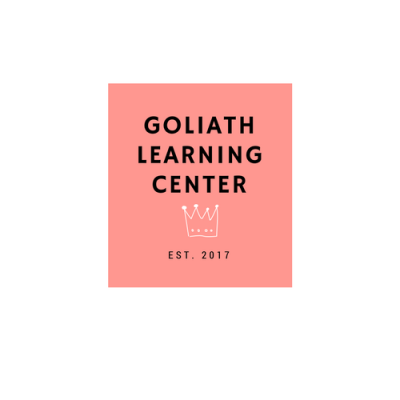Goliath Learning Center is an educational entity that offers an individualized learning experience.

## Middle School Math

• Whole Numbers
• Rational Numbers
• Integers
• Proportional Relationships
• Expressions
• Equations
• Algebraic Relationships
• Equations and Inequalities
• Coordinates/Graph Points
• Whole Numbers
• Rational Numbers
• Integers
• Proportional Relationships
• Expressions
• Equations
• Algebraic Relationships
• One-Variable Equations and Inequalities
• Coordinates/Graph Points
• Whole Numbers
• Real Numbers
• Rational Numbers
• Irrational Numbers
• Integers
• Proportional Relationships
• Expressions
• Equations
• Algebraic Relationships
• Slope
• Geometric Formulas
• Equations and Inequalities
• Coordinates/Graph Points (Rotation, Translation, Reflections, Dilations)